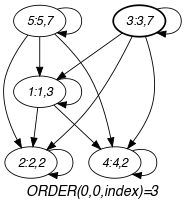## 5.242. max_index

Origin

N. Beldiceanu

Constraint

$\mathrm{𝚖𝚊𝚡}_\mathrm{𝚒𝚗𝚍𝚎𝚡}\left(\mathrm{𝙼𝙰𝚇}_\mathrm{𝙸𝙽𝙳𝙴𝚇},\mathrm{𝚅𝙰𝚁𝙸𝙰𝙱𝙻𝙴𝚂}\right)$

Arguments
 $\mathrm{𝙼𝙰𝚇}_\mathrm{𝙸𝙽𝙳𝙴𝚇}$ $\mathrm{𝚍𝚟𝚊𝚛}$ $\mathrm{𝚅𝙰𝚁𝙸𝙰𝙱𝙻𝙴𝚂}$ $\mathrm{𝚌𝚘𝚕𝚕𝚎𝚌𝚝𝚒𝚘𝚗}\left(\mathrm{𝚒𝚗𝚍𝚎𝚡}-\mathrm{𝚒𝚗𝚝},\mathrm{𝚟𝚊𝚛}-\mathrm{𝚍𝚟𝚊𝚛}\right)$
Restrictions
 $|\mathrm{𝚅𝙰𝚁𝙸𝙰𝙱𝙻𝙴𝚂}|>0$ $\mathrm{𝙼𝙰𝚇}_\mathrm{𝙸𝙽𝙳𝙴𝚇}\ge 0$ $\mathrm{𝙼𝙰𝚇}_\mathrm{𝙸𝙽𝙳𝙴𝚇}\le |\mathrm{𝚅𝙰𝚁𝙸𝙰𝙱𝙻𝙴𝚂}|$ $\mathrm{𝚛𝚎𝚚𝚞𝚒𝚛𝚎𝚍}$$\left(\mathrm{𝚅𝙰𝚁𝙸𝙰𝙱𝙻𝙴𝚂},\left[\mathrm{𝚒𝚗𝚍𝚎𝚡},\mathrm{𝚟𝚊𝚛}\right]\right)$ $\mathrm{𝚅𝙰𝚁𝙸𝙰𝙱𝙻𝙴𝚂}.\mathrm{𝚒𝚗𝚍𝚎𝚡}\ge 1$ $\mathrm{𝚅𝙰𝚁𝙸𝙰𝙱𝙻𝙴𝚂}.\mathrm{𝚒𝚗𝚍𝚎𝚡}\le |\mathrm{𝚅𝙰𝚁𝙸𝙰𝙱𝙻𝙴𝚂}|$ $\mathrm{𝚍𝚒𝚜𝚝𝚒𝚗𝚌𝚝}$$\left(\mathrm{𝚅𝙰𝚁𝙸𝙰𝙱𝙻𝙴𝚂},\mathrm{𝚒𝚗𝚍𝚎𝚡}\right)$
Purpose

$\mathrm{𝙼𝙰𝚇}_\mathrm{𝙸𝙽𝙳𝙴𝚇}$ is one of the indices of the collection of variables $\mathrm{𝚅𝙰𝚁𝙸𝙰𝙱𝙻𝙴𝚂}$ corresponding to its maximum value.

Example
$\left(\begin{array}{c}3,〈\begin{array}{cc}\mathrm{𝚒𝚗𝚍𝚎𝚡}-1\hfill & \mathrm{𝚟𝚊𝚛}-3,\hfill \\ \mathrm{𝚒𝚗𝚍𝚎𝚡}-2\hfill & \mathrm{𝚟𝚊𝚛}-2,\hfill \\ \mathrm{𝚒𝚗𝚍𝚎𝚡}-3\hfill & \mathrm{𝚟𝚊𝚛}-7,\hfill \\ \mathrm{𝚒𝚗𝚍𝚎𝚡}-4\hfill & \mathrm{𝚟𝚊𝚛}-2,\hfill \\ \mathrm{𝚒𝚗𝚍𝚎𝚡}-5\hfill & \mathrm{𝚟𝚊𝚛}-7\hfill \end{array}〉\hfill \end{array}\right)$

The attribute $\mathrm{𝚟𝚊𝚛}=7$ of the third and fifth items of the collection $\mathrm{𝚅𝙰𝚁𝙸𝙰𝙱𝙻𝙴𝚂}$ is the maximum value over values $3,2,7,2,7$. Consequently, the $\mathrm{𝚖𝚊𝚡}_\mathrm{𝚒𝚗𝚍𝚎𝚡}$ constraint holds since its first argument $\mathrm{𝙼𝙰𝚇}_\mathrm{𝙸𝙽𝙳𝙴𝚇}$ is set to $3\in \left\{3,5\right\}$.

Typical
 $|\mathrm{𝚅𝙰𝚁𝙸𝙰𝙱𝙻𝙴𝚂}|>0$ $\mathrm{𝚛𝚊𝚗𝚐𝚎}$$\left(\mathrm{𝚅𝙰𝚁𝙸𝙰𝙱𝙻𝙴𝚂}.\mathrm{𝚟𝚊𝚛}\right)>1$
Symmetries
• Items of $\mathrm{𝚅𝙰𝚁𝙸𝙰𝙱𝙻𝙴𝚂}$ are permutable.

• One and the same constant can be added to the $\mathrm{𝚟𝚊𝚛}$ attribute of all items of $\mathrm{𝚅𝙰𝚁𝙸𝙰𝙱𝙻𝙴𝚂}$.

Keywords
Arc input(s)

$\mathrm{𝚅𝙰𝚁𝙸𝙰𝙱𝙻𝙴𝚂}$

Arc generator
$\mathrm{𝐶𝐿𝐼𝑄𝑈𝐸}$$↦\mathrm{𝚌𝚘𝚕𝚕𝚎𝚌𝚝𝚒𝚘𝚗}\left(\mathrm{𝚟𝚊𝚛𝚒𝚊𝚋𝚕𝚎𝚜}\mathtt{1},\mathrm{𝚟𝚊𝚛𝚒𝚊𝚋𝚕𝚎𝚜}\mathtt{2}\right)$

Arc arity
Arc constraint(s)
$\bigvee \left(\begin{array}{c}\mathrm{𝚟𝚊𝚛𝚒𝚊𝚋𝚕𝚎𝚜}\mathtt{1}.\mathrm{𝚔𝚎𝚢}=\mathrm{𝚟𝚊𝚛𝚒𝚊𝚋𝚕𝚎𝚜}\mathtt{2}.\mathrm{𝚔𝚎𝚢},\hfill \\ \mathrm{𝚟𝚊𝚛𝚒𝚊𝚋𝚕𝚎𝚜}\mathtt{1}.\mathrm{𝚟𝚊𝚛}>\mathrm{𝚟𝚊𝚛𝚒𝚊𝚋𝚕𝚎𝚜}\mathtt{2}.\mathrm{𝚟𝚊𝚛}\hfill \end{array}\right)$
Graph property(ies)
$\mathrm{𝐎𝐑𝐃𝐄𝐑}$$\left(0,0,\mathrm{𝚒𝚗𝚍𝚎𝚡}\right)=\mathrm{𝙼𝙰𝚇}_\mathrm{𝙸𝙽𝙳𝙴𝚇}$

Graph model

Parts (A) and (B) of Figure 5.242.1 respectively show the initial and final graph associated with the Example slot. Since we use the $\mathrm{𝐎𝐑𝐃𝐄𝐑}$ graph property, the vertex of rank 0 (without considering the loops) of the final graph is outlined with a thick circle.

##### Figure 5.242.1. Initial and final graph of the $\mathrm{𝚖𝚊𝚡}_\mathrm{𝚒𝚗𝚍𝚎𝚡}$ constraint(a) (b)